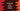# How to print Floyd's triangle in JavaScript in 3 ways## How to print Floyd’s triangle in JavaScript:

In this post, we will learn how to print Floyd’s triangle in JavaScript with examples. Before we start writing the program, let’s learn what is Floyd’s triangle and how to draw it programmatically.

### Floyd’s triangle:

Floyd’s triangle is a right-angled triangle of natural numbers. It starts with 1 in the top right corner and consecutive numbers for other numbers of the rows. For example:

``````1
2 3
4 5 6
7 8 9 10``````

To print Floyd’s triangle programmatically in JavaScript, we can use the following algorithm:

### Algorithm to print Floyd’s triangle:

To print Floyd’s triangle, the program will use two loops. One will run inside another. The outer loop will point to each row of the triangle and the inner loop will print the numbers.

• Take the height of the triangle and assign it to a variable.
• Create one variable as 1. This variable will be used to print the pattern numbers.
• Run one for loop from i = 1 to i = height.
• On each iteration, run another loop from j = 1 to j = i. On each iteration of the inner loop, print the variable created in the second step above.
• Increment the variable by 1 at the end of each iteration of the inner loop. Also, print a new line to move the control to the next row at the end of each iteration of the outer loop.

### JavaScript program to print Floyd’s triangle using for loop:

The following program prints Floyd’s triangle:

``````const size = 5;
let k = 1;

for (let i = 1; i <= size; i++) {
for (let j = 1; j <= i; j++) {
process.stdout.write(`\${k} `);
k++;
}
console.log();
}``````

Here,

• The size variable holds the height of the triangle, k is the value to print.
• The outer for-loop runs from i = 1 to i = size. On each iteration of the outer loop, the inner loop runs from j = 1 to j = i.
• The value of k is incremented by 1 on each iteration of the inner loop.
• We are using process.stdout.write to print the value of k as it doesn’t add a new line to the end. console.log is used to print a new line.

That’s all. If you run this program, it will print the below output:

``````1
2 3
4 5 6
7 8 9 10
11 12 13 14 15``````

The program is printing the triangle but the numbers are not aligned vertically. To fix this, we can use the padEnd method to append blank spaces to the end of a string to create a final string of equal predefined length.

Let’s try this:

``````const size = 5;
let k = 1;

for (let i = 1; i <= size; i++) {
for (let j = 1; j <= i; j++) {
k++;
}
console.log();
}``````

It will always create a string of length 3. So, for a single-digit number, it will add two spaces to the end and for a double-digit number, it will add one space.

### JavaScript program to print Floyd’s triangle using while loop:

We can use while loops to write the same example. Let’s write the above program with while loops:

``````const size = 10;
let k = 1,
i = 1,
j;

while (i <= size) {
j = 1;
while (j <= i) {
k++;
j++;
}
console.log();
i++;
}``````

Here, we used while loops to write the same algorithm. It will print the below result:

``````1
2  3
4  5  6
7  8  9  10
11 12 13 14 15
16 17 18 19 20 21
22 23 24 25 26 27 28
29 30 31 32 33 34 35 36
37 38 39 40 41 42 43 44 45
46 47 48 49 50 51 52 53 54 55 ``````

### JavaScript program to print Floyd’s triangle by using a separate function:

We can use a separate function to print the triangle. This function can take the height of the triangle as its parameter and print it out. It will separate the functionality from the main program.

``````function printFloydsTriangle(height) {
let k = 1;

for (let i = 1; i <= height; i++) {
for (let j = 1; j <= i; j++) {
k++;
}
console.log();
}
}

const height = 12;
printFloydsTriangle(height);``````

Here, we created a new function printFloydsTriangle to print Floyd’s triangle. It takes the height as its parameter and prints the triangle.

It will print:

``````1
2   3
4   5   6
7   8   9   10
11  12  13  14  15
16  17  18  19  20  21
22  23  24  25  26  27  28
29  30  31  32  33  34  35  36
37  38  39  40  41  42  43  44  45
46  47  48  49  50  51  52  53  54  55
56  57  58  59  60  61  62  63  64  65  66
67  68  69  70  71  72  73  74  75  76  77  78  ``````

You can adjust the padEnd parameter to change the spaces between the numbers of the triangle.